Quick Notes Free Energy (Gibbs)

• The feasibility of a reaction refers to whether it is allowed to happen.
• Feasible reactions can occur.
• Unfeasible reactions will never occur.
• Just because a reaction is feasible doesn't mean it will happen, only that it can - slow reaction rate and high activation barrier may prevent it from actually happening.
• For a reaction to be feasible, the Gibbs Free Energy content of a system must decrease (-ΔG).
• Gibbs Free Energy describes how much 'available' energy there is in a system.
• The change in Gibbs Free Energy (ΔG) of a reaction can be calculated:• where ΔH = enthalpy change, T = temperature (in Kelvin) and ΔS = entropy change.
• Units for entropy are based on Joules, this must be converted to kJ to match enthalpy.
• If for a reaction:
• -ΔH and +ΔS, the reaction is always feasible.
• -ΔH and -ΔS, the reaction may be feasible at low temperatures.
• +ΔH and +ΔS, the reaction may be feasible at high temperatures.
• +ΔH and -ΔS, the reaction is never feasible.
• The minimum temperature at which a reaction can occur is when ΔG = 0. Giving the equation:
•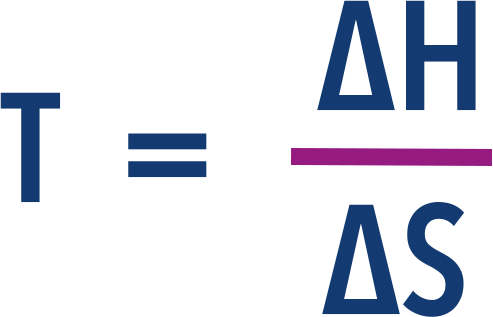Full Notes Free Energy (Gibbs)

A reaction that can happen, based on energy changes, is called feasible.

To determine whether a reaction is feasible, both enthalpy change and entropy change need to be considered.

Reactions are feasible if they lead to an increase in the stability of ‘the universe’. Essentially, energy must end up more spread out and less available in the universe after a reaction compared to before.

All reaction systems have 'enthalpy', this describes the internal energy of the system (see Enthalpy). This enthalpy is made up of energy that can flow out of the system as heat and entropy (the energy at a given temperature that can't flow out of the system as heat - see Entropy).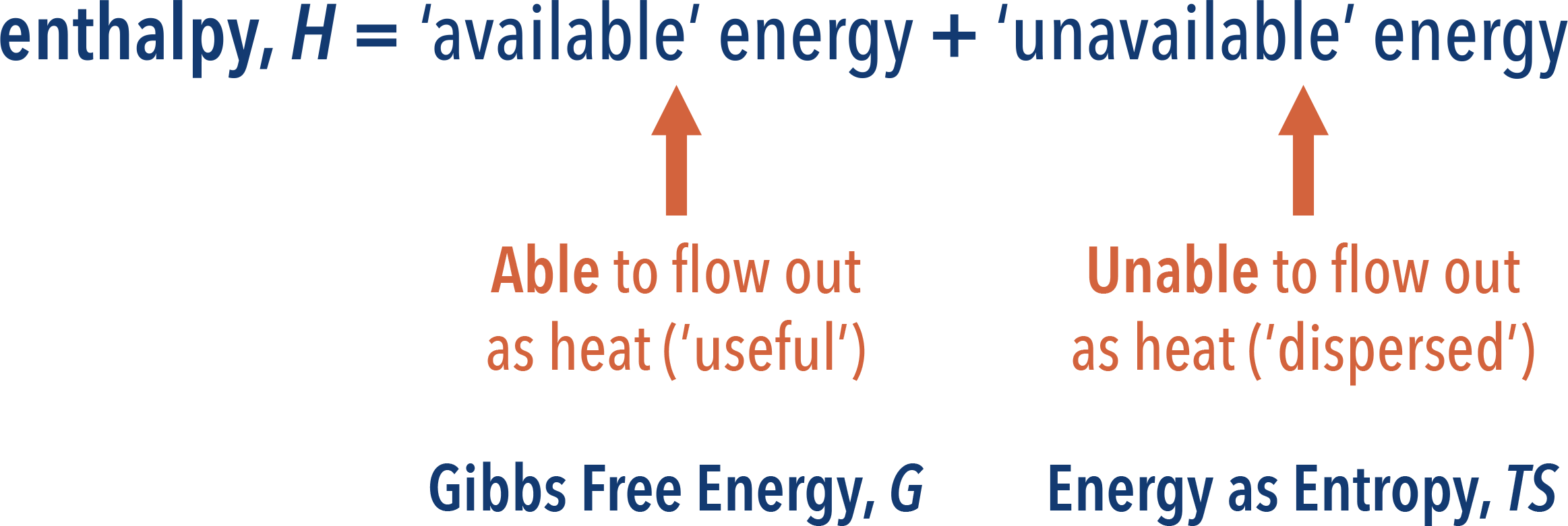The energy that can flow out as heat is called Gibbs Free Energy.

The greater the Gibbs Free Energy in a system, the less spread out the energy is. As energy has to end up more spread out after a reaction compared to before, for a reaction to be feasible, the amount of Gibbs Free Energy in a system has to decrease.

## Gibbs Free Energy Change, ΔGo

During a reaction, the enthalpy of a system changes. This means the Gibbs Free Energy and entropy content of the system must be changing as the enthalpy content is made up of them both.

The total enthalpy change of a system is made up of the change in Gibbs Free Energy and the change in energy as entropy (TΔS).If Gibbs Free Energy decreases (has a negative change, -ΔGo), this means energy is ending up more spread out in the universe in some way and the reaction is feasible (see above).

The Gibbs Free Energy Change, ΔGo of a reaction can't be measured directly but if the enthalpy change and entropy change (and temperature) are known, the above equation can be rearranged to calculate it.Now, it is straightforward to see if the value for Gibbs Free Energy change, ΔG, will be negative or positive. We just need to subtract TΔS from ΔH. Be careful of units - see below.

Sometimes, it can be possible to determine whether ΔG will be negative or positive just be looking at the signs of the enthalpy and entropy changes.

For Example...

If a reaction has a negative enthalpy change (exothermic, -ΔH) and a positive entropy change (+ΔS), the reaction will always be feasible, as the free energy value will always be negative (-ΔG).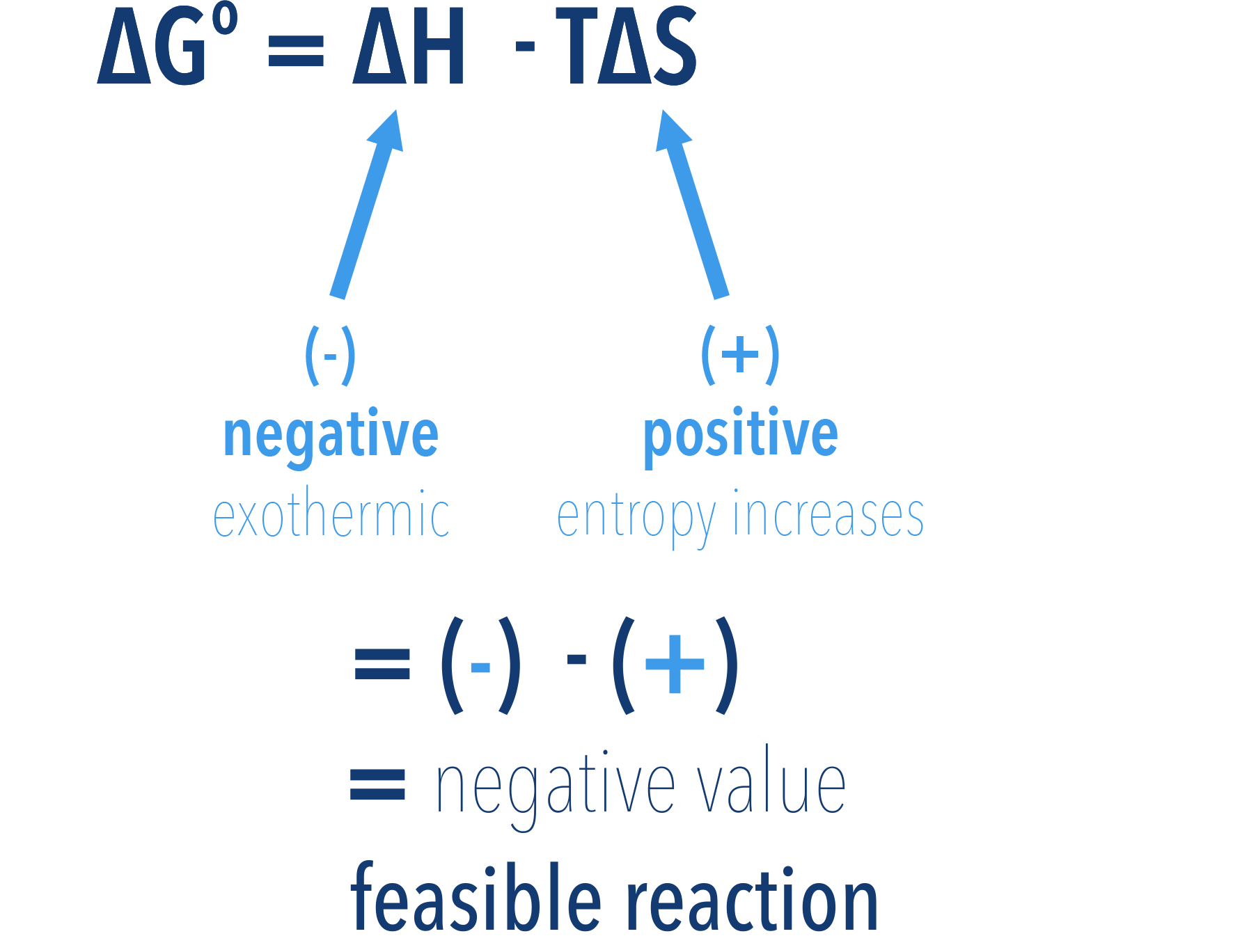If a reaction has a negative enthalpy change (exothermic, -ΔH) and a negative entropy change (-ΔS), the reaction can be feasible, but only at low temperatures.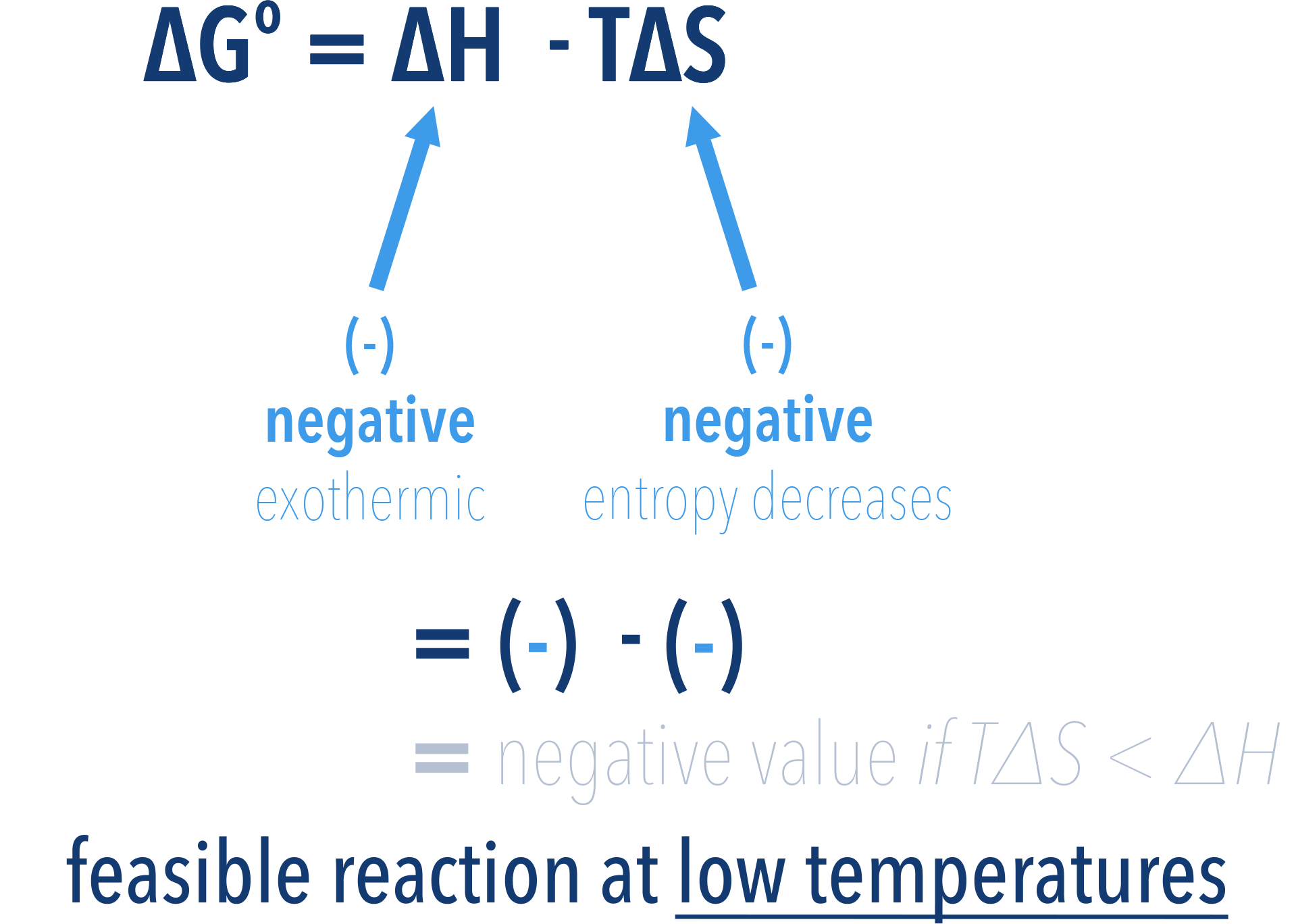If a reaction has a positive enthalpy change (endothermic, +ΔH) and a positive entropy change (+ΔS), the reaction can be feasible, but only at high temperatures.If a reaction has a positive enthalpy change (endothermic, +ΔH) and a negative entropy change (-ΔS), the reaction is never feasible, as the free energy value will always be greater than zero (+ΔG).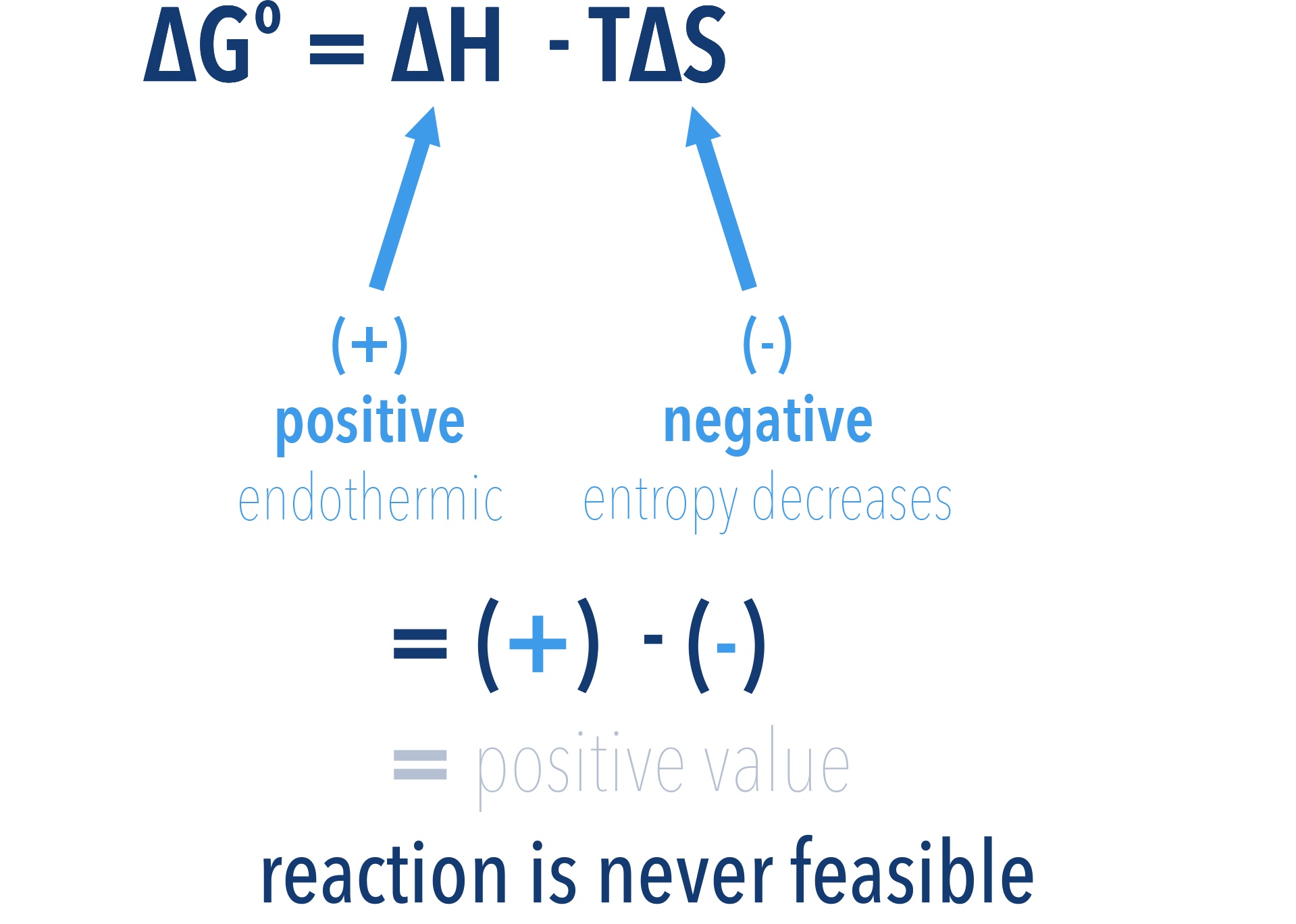## Determining the minimum temperature at which a reaction is feasible

As shown above, some reactions are only feasible above or below a certain temperature. This is because temperature, T, changes the TΔS part of the equation for Gibbs Free Energy.

A reaction can only be feasible if ΔG value is negative. This means the temperature at which ΔG is zero is the minimum temperature possible for the reaction to be feasible. Any higher and the ΔG value will become negative (feasible). Any smaller, and the ΔG value will be positive (unfeasible).

As a result, we can find this minimum temperature by rearranging and substituting 0 for ΔG into the above equation...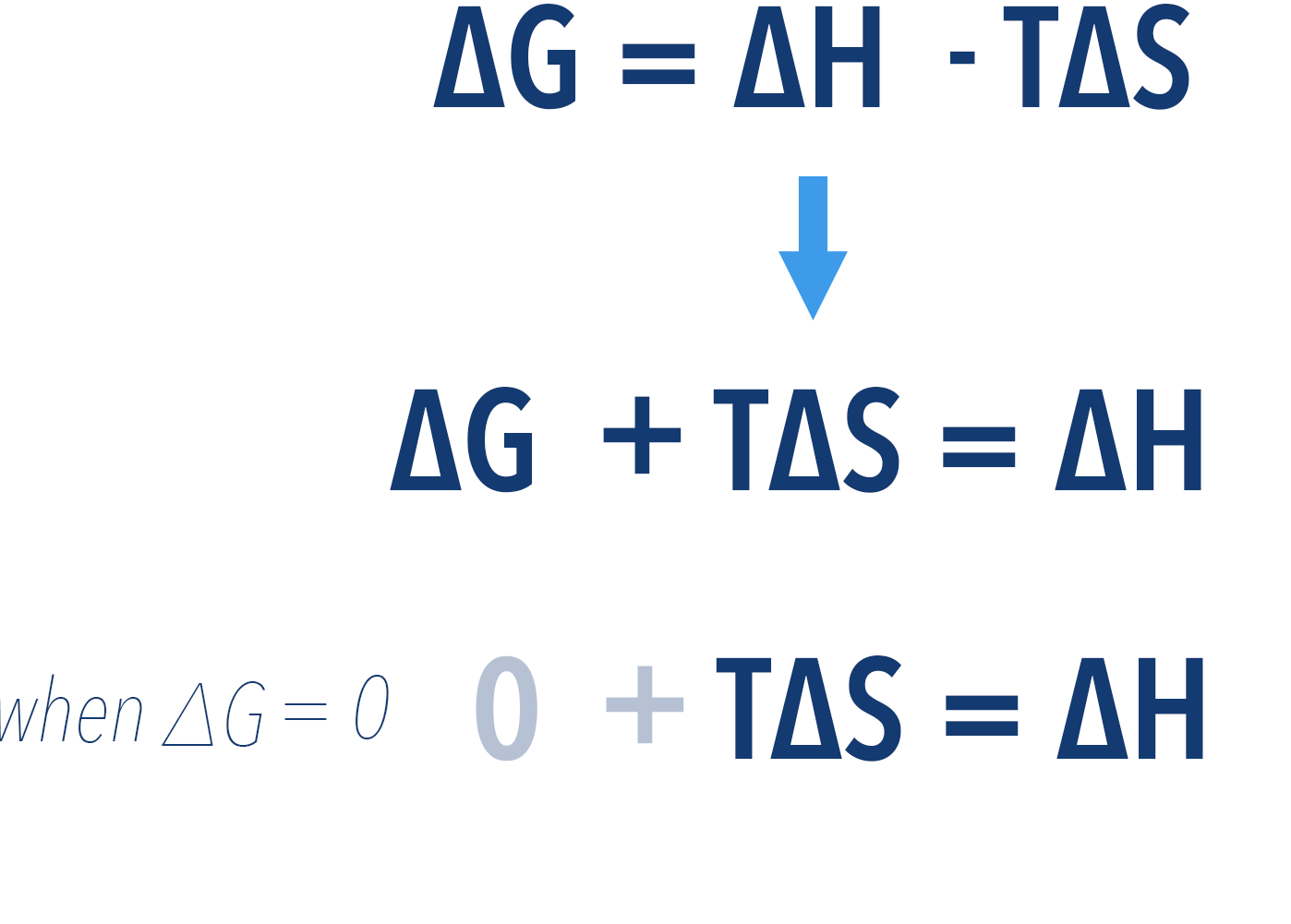Now, we can rearrange this again to find temperature...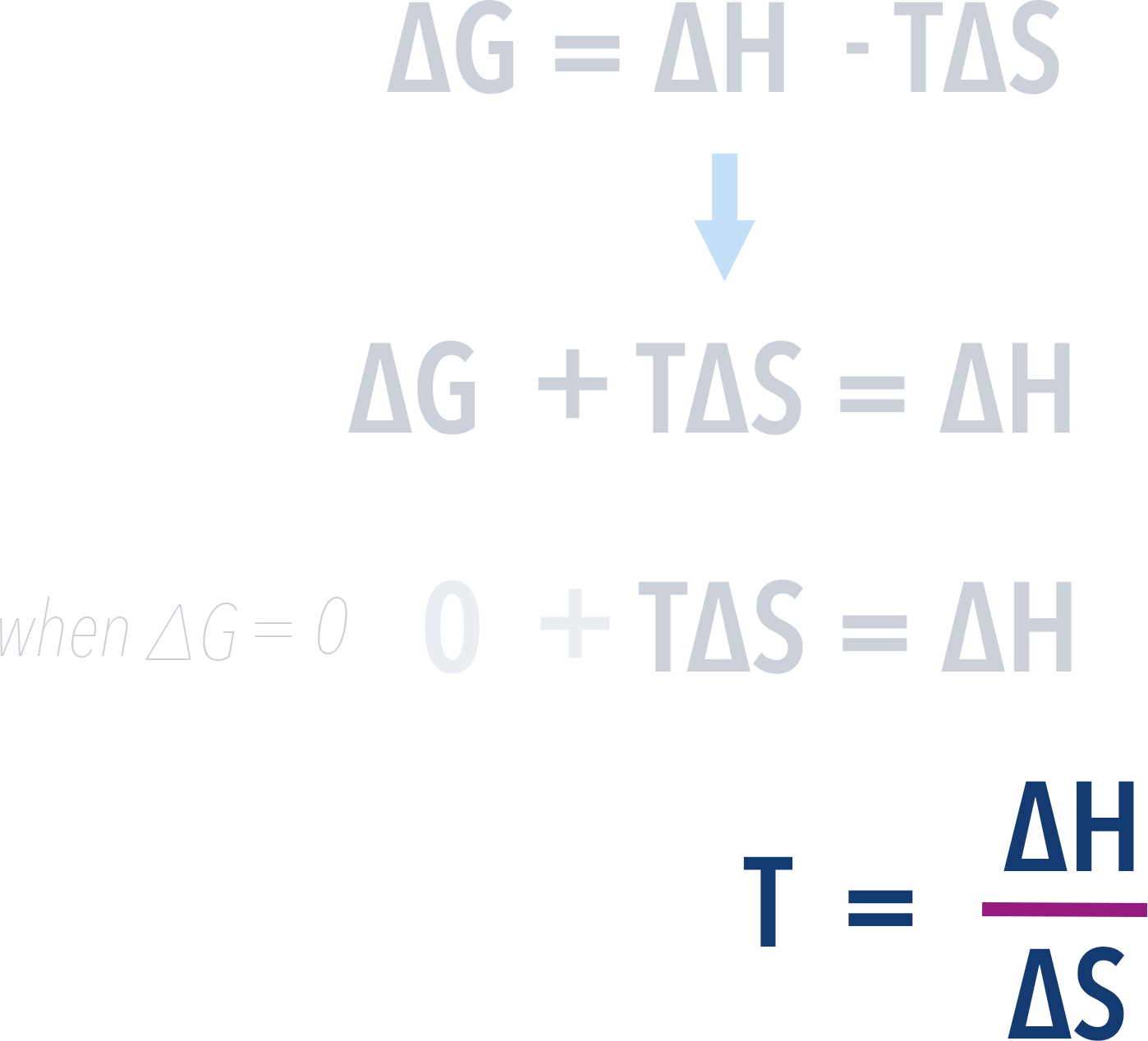When working with Gibbs Free Energy, it is important to remember the units. Entropy is given in J K-1 mol-1 and enthalpy in kJ mol-1. For calculations, convert entropy into kJ before finding the free energy change.

## Why do some feasible reactions not happen?

The feasibility of a reaction is based on the energy changes (enthalpy, entropy and Gibbs Free Energy) that would occur during the reaction. It is a way of describing whether a reaction is 'allowed' to happen, not whether it will happen.

All students are 'allowed' to get 100% in an exam, this doesn't mean they all will!

Very slow rates of reaction and high activation energy barriers can prevent feasible reactions from occuring.

## What is the difference between spontaneous and feasible?

The terms feasible and spontaneous are often both used at this level to describe reactions that are allowed occur. Used in this way, there is no difference between the two.

I prefer the term feasible, as spontaneous in every day language implies the reaction will just happen by itself instantly (think of spontaneous combustion!) and that isn't the correct meaning here.